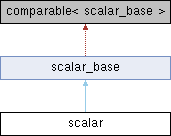Qpid Proton C++ API 0.39.0
Searching...
No Matches

A holder for an instance of any scalar AMQP type. More...

`#include <scalar.hpp>`

Inheritance diagram for scalar:## Public Member Functions

scalar ()=default
Create an empty scalar.

template<class T >
scalar (const T &x)
Construct from any scalar type.

template<class T >
scalaroperator= (const T &x)
Assign from any scalar type.

void clear ()
Clear the scalar, making it empty().Public Member Functions inherited from scalar_base
type_id type () const
AMQP type of data stored in the scalar.

bool empty () const
True if there is no value, i.e. type() == NULL_TYPE.

## Related Functions

(Note that these are not member functions.)

template<class T >
get (const scalar &s)
Get a contained value of type T. More...

template<class T >
coerce (const scalar &x)
Coerce the contained value to type T. More...

template<class T >
coerce (scalar &x)
Coerce the contained value to type T. More...

## Detailed Description

A holder for an instance of any scalar AMQP type.

AMQP and C++ types

## ◆ get()

 T get ( const scalar & s )
related

Get a contained value of type T.

For example:

``` uint64_t i = get<uint64_t>(x)
```

This will succeed if and only if x contains a uint64_t value.

Exceptions
 conversion_error if contained value is not of type T.

## ◆ coerce() [1/2]

 T coerce ( const scalar & x )
related

Coerce the contained value to type T.

For example:

``` uint64_t i = coerce<uint64_t>(x)
```

This will succeed if x contains any numeric value, but may lose precision if it contains a float or double value.

Exceptions
 conversion_error if the value cannot be converted to T according to `std::is_convertible`

## ◆ coerce() [2/2]

 T coerce ( scalar & x )
related

Coerce the contained value to type T.

For example:

``` uint64_t i = coerce<uint64_t>(x)
```

This will succeed if x contains any numeric value, but may lose precision if it contains a float or double value.

Exceptions
 conversion_error if the value cannot be converted to T according to `std::is_convertible`

The documentation for this class was generated from the following file: